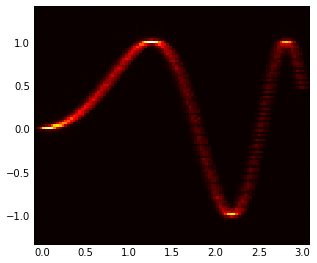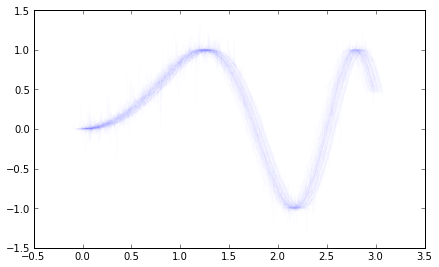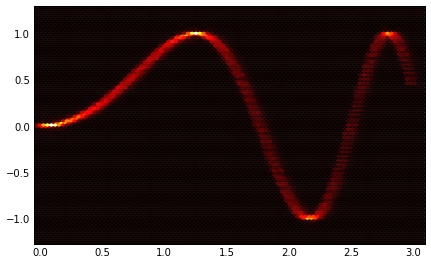# Eye patterns (Heat plot?)

I'm using matplotlib from pylab to generate eye patterns for signal
simulations. My output pretty much looks like this:

http://www.flickr.com/photos/31208937@...3933.../6079131690/

Its pretty useful as it allows one to quickly see the size of the eye
opening, the maximum/minimum voltage, etc. I'd really like to be able
to create a heat diagram, like these:

Is there any way within matplotlib to do that right now?

From: Russ Dill [mailto:russ.dill@…287…]
Sent: Saturday, January 21, 2012 16:31

I'm using matplotlib from pylab to generate eye patterns for signal
simulations.

...

Is there any way within matplotlib to do that right now?

One way combines Numpy's histogram2d and matplotlib's imshow, as in the
example in the histogram2d docs . The example's x array should become all
of the time samples in your traces, strung together in one dimension; the y
array, the corresponding voltage samples.

If you have certain structure, such as a regular and consistent grid of times,
you might instead construct a series of vertical, 1-d histograms (paying
attention to normalization); column-stack them into the final 2-d histogram;
and again plot with imshow.

I'll try it out and see what I get, but I don't think it will work so
well. The problem is that while the data is made up of x/y samples, it
actually represents a line. The samples should be evenly distributed
not along the x or y axis, but along the length of the line. I feel
like I'll need a line drawing algorithm.

(For example, if samples are evenly distributed along the x axis, a 89
degree line is highly under-represented, but a 1 degree line is highly
over-represented. The number of samples should be sqrt(dx^2 + dy^2),
but with evenly spaced x samples, its just dx.

···

On Mon, Jan 23, 2012 at 11:17 AM, Stan West <stan.west@...706...> wrote:

From: Russ Dill [mailto:russ.dill@…287…]
Sent: Saturday, January 21, 2012 16:31

I'm using matplotlib from pylab to generate eye patterns for signal
simulations.

...

Is there any way within matplotlib to do that right now?

One way combines Numpy's histogram2d and matplotlib's imshow, as in the
example in the histogram2d docs . The example's x array should become all
of the time samples in your traces, strung together in one dimension; the y
array, the corresponding voltage samples.

I don't know of a way to directly produce the LeCroy heatmap in Python, so here's my idea for a hack:
*Each sample point you have from the trace represents a point in XY coordinates.
*Similarly, the plot area is filled with regularly spaced XY coordinates.
*Every trace sample will fall within a square bounding box with four points.
*Each plot area point gets a membership value, based on distance between centers of the sample point and the plot area point.
*To construct the heat diagram, sum the membership values of all sample points for all traces.
*Display it with a contour plot, but without the isovalue lines.

-Ethan

···

On 1/23/2012 1:55 PM, Russ Dill wrote:

On Mon, Jan 23, 2012 at 11:17 AM, Stan West<stan.west@...706...> wrote:

From: Russ Dill [mailto:russ.dill@…287…]
Sent: Saturday, January 21, 2012 16:31

I'm using matplotlib from pylab to generate eye patterns for signal
simulations.

...

Is there any way within matplotlib to do that right now?

One way combines Numpy's histogram2d and matplotlib's imshow, as in the
example in the histogram2d docs . The example's x array should become all
of the time samples in your traces, strung together in one dimension; the y
array, the corresponding voltage samples.

I'll try it out and see what I get, but I don't think it will work so
well. The problem is that while the data is made up of x/y samples, it
actually represents a line. The samples should be evenly distributed
not along the x or y axis, but along the length of the line. I feel
like I'll need a line drawing algorithm.

(For example, if samples are evenly distributed along the x axis, a 89
degree line is highly under-represented, but a 1 degree line is highly
over-represented. The number of samples should be sqrt(dx^2 + dy^2),
but with evenly spaced x samples, its just dx.

matplotlib also has hexbin() if that helps.

http://matplotlib.sourceforge.net/api/axes_api.html?highlight=hexbin#matplotlib.axes.Axes.hexbin

Ben Root

···

On Mon, Jan 23, 2012 at 1:46 PM, Ethan Swint <eswint@…3245…> wrote:

On 1/23/2012 1:55 PM, Russ Dill wrote:

On Mon, Jan 23, 2012 at 11:17 AM, Stan West<stan.west@…706…> wrote:

From: Russ Dill [mailto:russ.dill@…287…]

Sent: Saturday, January 21, 2012 16:31

I’m using matplotlib from pylab to generate eye patterns for signal

simulations.

Is there any way within matplotlib to do that right now?

One way combines Numpy’s histogram2d and matplotlib’s imshow, as in the

example in the histogram2d docs . The example’s x array should become all

of the time samples in your traces, strung together in one dimension; the y

array, the corresponding voltage samples.

I’ll try it out and see what I get, but I don’t think it will work so

well. The problem is that while the data is made up of x/y samples, it

actually represents a line. The samples should be evenly distributed

not along the x or y axis, but along the length of the line. I feel

like I’ll need a line drawing algorithm.

(For example, if samples are evenly distributed along the x axis, a 89

degree line is highly under-represented, but a 1 degree line is highly

over-represented. The number of samples should be sqrt(dx^2 + dy^2),

but with evenly spaced x samples, its just dx.

I don’t know of a way to directly produce the LeCroy heatmap in Python,

so here’s my idea for a hack:

*Each sample point you have from the trace represents a point in XY

coordinates.

*Similarly, the plot area is filled with regularly spaced XY coordinates.

*Every trace sample will fall within a square bounding box with four points.

*Each plot area point gets a membership value, based on distance between

centers of the sample point and the plot area point.

*To construct the heat diagram, sum the membership values of all sample

points for all traces.

*Display it with a contour plot, but without the isovalue lines.

-Ethan

Hi Russ,

Russ Dill, on 2012-01-21 13:30, wrote:

I'm using matplotlib from pylab to generate eye patterns for signal
simulations. My output pretty much looks like this:

http://www.flickr.com/photos/31208937@...3933.../6079131690/

Its pretty useful as it allows one to quickly see the size of the eye
opening, the maximum/minimum voltage, etc. I'd really like to be able
to create a heat diagram, like these:

Is there any way within matplotlib to do that right now?

the quick and dirty way to get close to what you want is to add
an alpha value to the lines you're already plotting. Here's a
small example:

x = np.arange(0,3,.01)
y = np.sin(x**2)
all_x,all_y = [],[]
ax = plt.gca()
for i in range(100):
noisex = np.random.randn(1)*.04
noisey = (np.random.randn(x.shape)*.2)**3
ax.plot(x+noisex,y+noisey, color='b', alpha=.01)
all_x.append(x+noisex)
all_y.append(y+noisey)

To get a heat diagram, as was suggested, you can use a 2d
histogram.

plt.figure()
all_x =np.array(all_x)
all_y = np.array(all_y)
all_x.shape = all_y.shape = -1
H, yedges, xedges = np.histogram2d(all_y, all_x, bins=100)
extent = [xedges, xedges[-1], yedges[-1], yedges]
ax = plt.gca()
plt.hot()
ax.imshow(H, extent=extent, interpolation='nearest')
ax.invert_yaxis()

I'm attaching the two images for reference

best,···

--
Paul Ivanov
314 address only used for lists, off-list direct email at:
http://pirsquared.org | GPG/PGP key id: 0x0F3E28F7

Paul Ivanov, on 2012-01-23 13:07, wrote:

the quick and dirty way to get close to what you want is to add
an alpha value to the lines you're already plotting. Here's a
small example:

x = np.arange(0,3,.01)
y = np.sin(x**2)
all_x,all_y = [],[]
ax = plt.gca()
for i in range(100):
noisex = np.random.randn(1)*.04
noisey = (np.random.randn(x.shape)*.2)**3
ax.plot(x+noisex,y+noisey, color='b', alpha=.01)
all_x.append(x+noisex)
all_y.append(y+noisey)

To get a heat diagram, as was suggested, you can use a 2d
histogram.

plt.figure()
all_x =np.array(all_x)
all_y = np.array(all_y)
all_x.shape = all_y.shape = -1
H, yedges, xedges = np.histogram2d(all_y, all_x, bins=100)
extent = [xedges, xedges[-1], yedges[-1], yedges]
ax = plt.gca()
plt.hot()
ax.imshow(H, extent=extent, interpolation='nearest')
ax.invert_yaxis()

For completeness, attached is what the hexbin version of the same
data looks like:

plt.hexbin(all_x, all_y)

You may want to play with the 'bins' (for histogram2d) and
'griddata' (for hexbin) parameters to get the appropriate level
of detail for the amount of data you have.

best,···

--
Paul Ivanov
314 address only used for lists, off-list direct email at:
http://pirsquared.org | GPG/PGP key id: 0x0F3E28F7

To get a proper count of the 2D bins that each curve crosses, you could parameterize the curve by arclength and resample it use a small step size. Or, you could linearly interpolate between the curve’s discretized data points using Bresenham’s line algorithm. The latter seemed like a straightforward approach, so I wrote it up and added it to the SciPy Cookbook:

``````[http://www.scipy.org/Cookbook/EyeDiagram](http://www.scipy.org/Cookbook/EyeDiagram)
``````

Warren

···

On Mon, Jan 23, 2012 at 3:19 PM, Paul Ivanov <pivanov314@…287…> wrote:

Paul Ivanov, on 2012-01-23 13:07, wrote:

the quick and dirty way to get close to what you want is to add

an alpha value to the lines you’re already plotting. Here’s a

small example:

x = np.arange(0,3,.01)

y = np.sin(x**2)

all_x,all_y = [],[]

ax = plt.gca()

for i in range(100):

``````  noisex = np.random.randn(1)*.04
``````
``````  noisey = (np.random.randn(x.shape)*.2)**3
``````
``````  ax.plot(x+noisex,y+noisey, color='b', alpha=.01)
``````
``````  all_x.append(x+noisex)
``````
``````  all_y.append(y+noisey)
``````

To get a heat diagram, as was suggested, you can use a 2d

histogram.

plt.figure()

all_x =np.array(all_x)

all_y = np.array(all_y)

all_x.shape = all_y.shape = -1

H, yedges, xedges = np.histogram2d(all_y, all_x, bins=100)

extent = [xedges, xedges[-1], yedges[-1], yedges]

ax = plt.gca()

plt.hot()

ax.imshow(H, extent=extent, interpolation=‘nearest’)

ax.invert_yaxis()

For completeness, attached is what the hexbin version of the same

data looks like:

plt.hexbin(all_x, all_y)

You may want to play with the ‘bins’ (for histogram2d) and

‘griddata’ (for hexbin) parameters to get the appropriate level

of detail for the amount of data you have.